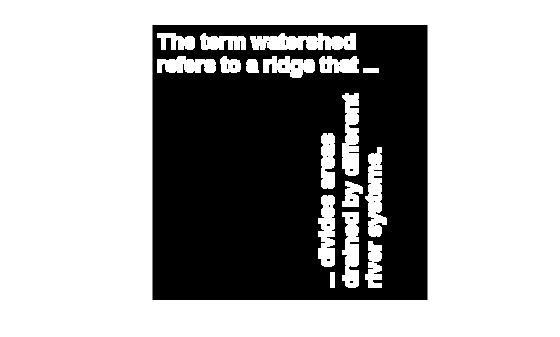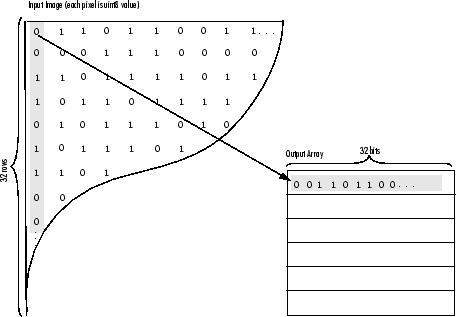# bwpack

Pack binary image

## Syntax

``BWP = bwpack(BW)``

## Description

example

````BWP = bwpack(BW)` packs the binary image `BW` into the `uint32` array `BWP`, which is known as a packed binary image. Because each pixel value in the binary image has only two possible values, `1` and `0`, `bwpack` can map each pixel to a single bit in the packed output image.```

## Examples

collapse all

Read binary image into the workspace.

```BW = imread('text.png'); imshow(BW)```Pack the image.

`BWp = bwpack(BW);`

Dilate the packed image.

`BWp_dilated = imdilate(BWp,ones(3,3),'ispacked');`

Unpack the dilated image and display it.

```BW_dilated = bwunpack(BWp_dilated, size(BW,1)); imshow(BW_dilated)```## Input Arguments

collapse all

Binary image, specified as a 2-D numeric or logical matrix. For numeric input, any nonzero pixels are considered to be `1` (`true`).

Data Types: `single` | `double` | `int8` | `int16` | `int32` | `int64` | `uint8` | `uint16` | `uint32` | `uint64` | `logical`

## Output Arguments

collapse all

Packed binary image, returned as a numeric matrix of type `uint32`.

Data Types: `uint32`

## Tips

• Binary image packing is used to accelerate some binary morphological operations, such as dilation and erosion. If the input to `imdilate` or `imerode` is a packed binary image, then the function uses a specialized routine to perform the operation faster.

• Use `bwunpack` to unpack packed binary images.

## Algorithms

`bwpack` processes the input image pixels by column, mapping groups of 32 pixels into the bits of a `uint32` value. The first pixel in the first row corresponds to the least significant bit of the first `uint32` element of the output array. The first pixel in the 32nd input row corresponds to the most significant bit of this same element. The first pixel of the 33rd row corresponds to the least significant bit of the second output element, and so on. If `BW` is `M`-by-`N`, then `BWP` is `ceil(M/32)-by-N`. This figure illustrates how `bwpack` maps the pixels in a binary image to the bits in a packed binary image.